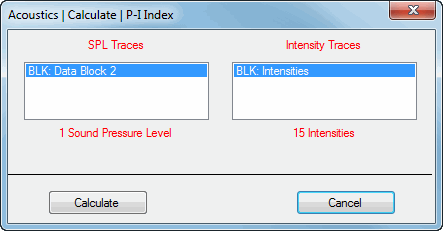Acoustics | Calculate | P-I Index

Calculates the Pressure-Intensity (P-I) Index from SPL and Intensity measurement functions.  The P-I Index is defined as,

P-I Index (dB) = (SPL (dB Reference 20 m PA) - Intensity (dB Reference 1e-12 watts/m2) )

When this command is executed, a dialog box is opened.When Calculate is pressed, a new Data Block file with P-I Index measurements in it will be created.